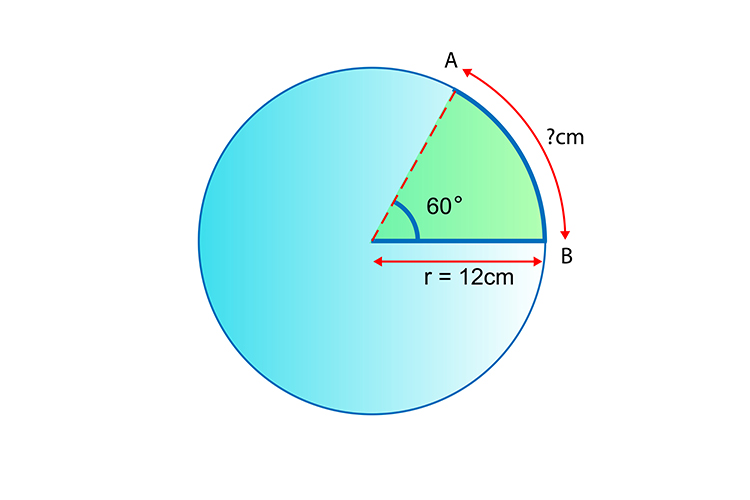# Arc of a sector

Using the same reasoning about radians we can also work out the length of any arc on a circle.

Example

Work out the length of the arc ABCircumference=2pir

Therefore Circumference=2pi times12 cm

=24pi cm

Logic progresses as follows:

24pi=100%of the circumference

360^@=24pi cm

60^@=x cm

 360/60 = (24pi)/x x = (60times24pi)/360 x = (1times24pi)/6 x = 4pi

Answer: The arc is x=4pi cm long